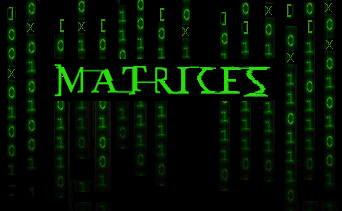Search IntMath
Close

450+ Math Lessons written by Math Professors and Teachers

5 Million+ Students Helped Each Year

1200+ Articles Written by Math Educators and Enthusiasts

Simplifying and Teaching Math for Over 23 Years

# Matrices and Determinants

By M Bourne

## Why study the Matrix...?

A matrix is simply a set of numbers arranged in a rectangular table.

((2,4,-1,0),(1,3,7,2))

On the right is an example of a 2 × 4 matrix. It has 2 rows and 4 columns. We usually write matrices inside parentheses ( ) or brackets [ ].

We can add, subtract and multiply matrices together, under certain conditions.

We use matrices to solve simultaneous equations, that we met earlier. Matrices are used to solve problems in:• electronics
• statics
• robotics
• linear programming
• optimisation
• intersections of planes
• genetics

We see several of these applications throughout this chapter, especially in Matrices and Linear Equations.

For large systems of equations, we use a computer to find the solution. This chapter first shows you the basics of matrix arithmetic, and then we show some computer examples (using Scientific Notebook or similar) so that you understand what the computer is doing for you.

You can skip over the next part if you want to go straight to matrices.

## Determinants

A determinant of a matrix represents a single number. We obtain this value by multiplying and adding its elements in a special way. We can use the determinant of a matrix to solve a system of simultaneous equations.

For example, if we have the (square) 2 × 2 matrix:

((5,7),(2,-3))

then the determinant of this matrix is written within vertical lines as follows:

|(5,7),(2,-3)|

We'll see in the next section how to evaluate this determinant. (It has value -29).

## In this Chapter

1. Determinants - derived from a square matrix, a determinant needs to be multiplied out to give a single number.

2. Large Determinants - this section will help you to understand smaller determinants.

3. Matrices - definition, features, identity matrix, and examples

4. Multiplication of Matrices - how to multiply matrices of different sizes. Includes an interactive where you can explore the concept.

5. Finding the Inverse of a Matrix - which we use to solve systems of equations

6. Matrices and Linear Equations - how to solve systems of equations with matrices

We begin the chapter with an introduction to Determinants »

## Problem SolverThis tool combines the power of mathematical computation engine that excels at solving mathematical formulas with the power of GPT large language models to parse and generate natural language. This creates math problem solver thats more accurate than ChatGPT, more flexible than a calculator, and faster answers than a human tutor. Learn More.